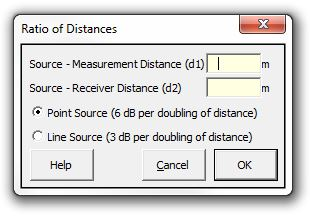### Strutt Help

Ratio of Distances    1/1, 1/3

Strutt|Propagation|Ratio of Distances, inserts the ratio of distances correction into the current row of the worksheet, to convert a measured sound level at a known distance (d_1 to a sound level at a given distance d_2)Strutt implements the formula as:

Delta L = Clog_10(d_2/d_1), where

C is a constant and has the value 10 for line source (3 dB per doubling of distance), and has the value 20 for point source (6 dB for doubling of distance).